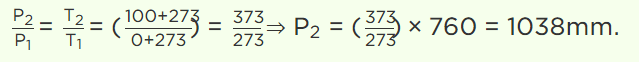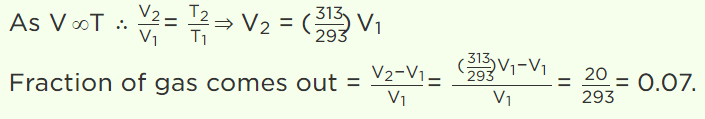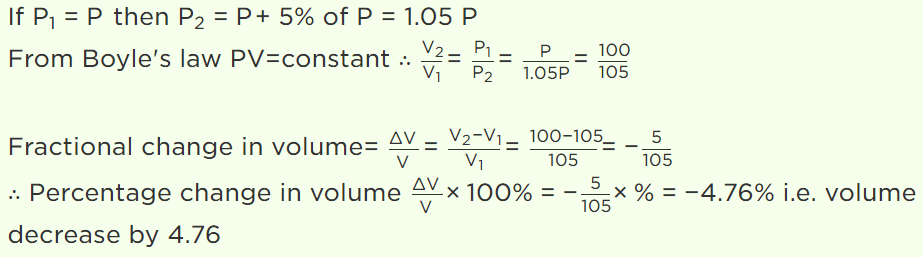## Kinetic Theory Questions and Answers Part-3

1. A cylinder of 5 litre capacity, filled with air at N.T.P. is connected with another evacuated cylinder of 30 litres of capacity. The resultant air pressure in both the cylinders will be
a) 38.85 cm of Hg
b) 21.85 cm of Hg
c) 10.85 cm of Hg
d) 14.85 cm of Hg

Explanation:2. Volume of gas become four times if
a) Temperature become four times at constant pressure
b) Temperature become one fourth at constant pressure
c) Temperature becomes two times at constant pressure
d) Temperature becomes half at constant pressure

Explanation: Volume of gas become four times if temperature become four times at constant pressure

3. The relationship between pressure and the density of a gas expressed by Boyle’s law, P = KD holds true
a) For any gas under any conditions
b) For some gases under any conditions
c) Only if the temperature is kept constant
d) Only if the density is constant

Explanation: Only if the temperature is kept constant

4. Kinetic theory of gases provide a base for
a) Charle’s law
b) Boyle’s law
c) Charle’s law and Boyle’s law
d) None of these

Explanation: Kinetic theory of gases provide a base for Charle’s law and Boyle’s law

5. On $0^{\circ} C$  pressure measured by barometer is 760 mm. What will be pressure on $100^{\circ} C$
a) 760 mm
b) 730 mm
c) 780 mm
d) None of these

Explanation:6. A perfect gas at $27^{\circ} C$  is heated at constant pressure to $327^{\circ} C$  . If original volume of gas (at $27^{\circ} C$  is V then volume at $327^{\circ} C$  is
a) V
b) 3 V
c) 2 V
d) V / 2

Explanation:7. Hydrogen gas is filled in a balloon at $20^{\circ} C$  . If temperature is made $40^{\circ} C$ , pressure remaining same, what fraction of Hydrogen will come out
a) 0.07
b) 0.25
c) 0.5
d) 0.75

Explanation:8. At constant temperature on increasing the pressure of a gas by 5% will decrease its volume by
a) 5%
b) 5.26%
c) 4.26%
d) 4.76%

Explanation:9. Which law states that effect of pressure is same for all portion
a) Pascal’s law
b) Gallusac’s law
c) Dalton’s law
d) None of these